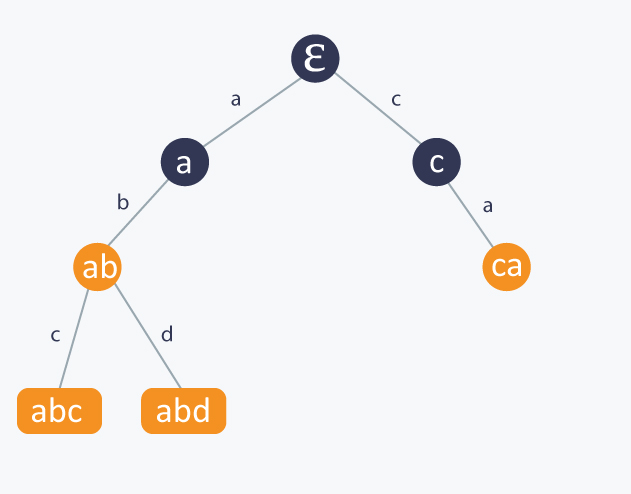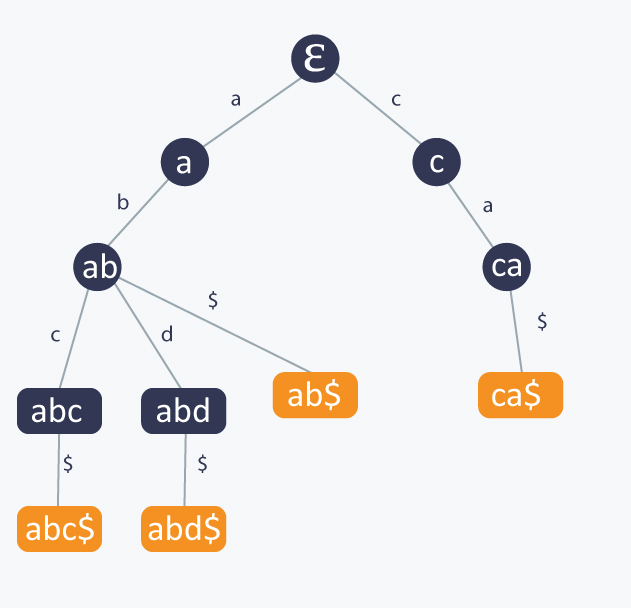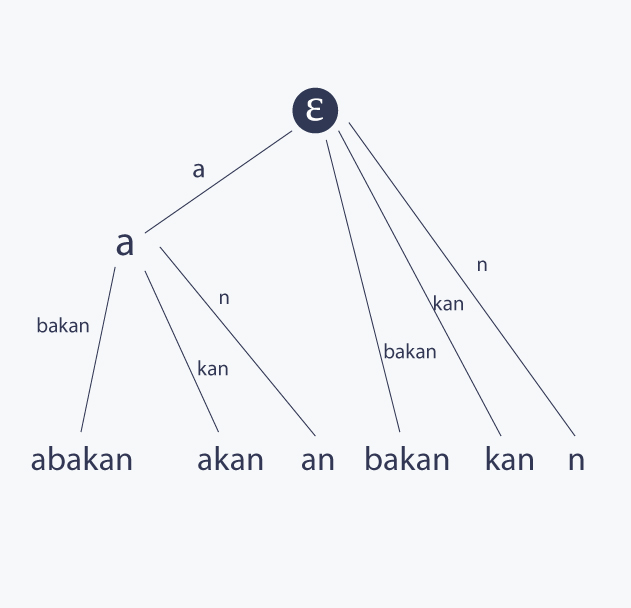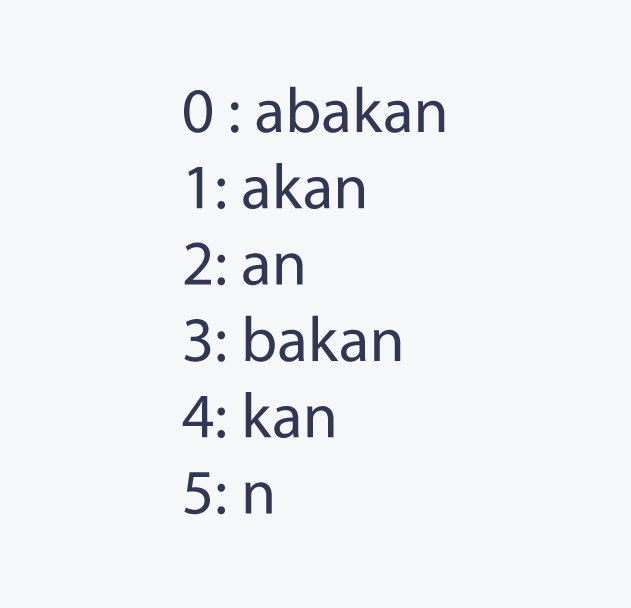56
Trie, Suffix Tree, Suffix Array
Suffix-array
Suffix-tree
Trie

In this Codemonk article, I am going to write about 3 very useful string data structures. The topic is very broad, and since all these data structures are very well studied and explained in external resources, the article will have a different format that previous ones. Rather than describing implementation details, I am going to provide a well structured overview about the topic, link related detailed descriptions to each structure, and give you some intuitions when to use which structure and what are advantages and tradeoffs while using each of them. From now Σ will denote the alphabet we working with, for example latin lowercase letters, and |Σ| will be the size of Σ.

### Trie

Trie is probably the most basic and intuitive tree based data structure designed to use with strings.

Let S be a set of k strings, in other words S = {s1, s2, ..., sk}. In addition, let P be a pattern we want to match with any of strings in S. The question is how to build a very basic tree based data structure, which allows us to decide if given P matches any string in S. How to model such a data structure? Well, we can model the set S as a rooted tree T in such a way, that each path from the root of T to any of its nodes, corresponds to a prefix of at least one string of S. The best way to present this construction, is to consider an example set. Let S = {abc, abd, ca, ab}. Let ε corresponds to an empty string. Then a trie for S looks like this:Notice that each edge of an internal node u to its child v is marked by a letter from our alphabet denoting the extension of string represented by u to a string represented by v. Moreover, each node corresponding to a string from S is marked orange. One observation is that if some si is a prefix of another string from S, then a node corresponding to si is an internal node of T, otherwise it is a leaf. Sometimes, it is useful to have all nodes corresponding to strings from S as leaves, and it is very common to append to each strings from S a character which is guaranteed not to be in Σ, in our case, we will denote \$ as this special character. Then such a modified trie from our example will look like this:Notice that since now there is no string in S which is a prefix another string from S, all nodes in T corresponding to strings from S are leaves.

Building a trie T is very simple. At the beginning, you start with just one node representing the empty string ε. If you want to insert a string s to T, you start at the root of T and consider the first character c of s. If there is an edge marked with c from the current node to any of its children, you consume the character c and get down to this child. If at some point there is no such an edge and child, you have to create them, consume c and continue the process until whole s is consumed.

Searching a string P in T is even simpler, you just have to iterate through the characters of P and follow corresponding edges to these characters in T starting from the root. If at some point there is no transition to a children, or if you consume all the letter of P, but the node in which you end the process does not correspond to any string from S, then P does not belong to S either. Otherwise, you end the process in a node corresponding to P, so we know that P belongs to S.

One other basic application of a trie is to decide if P is a substring of s, in other words, the pattern matching problem. How we can solve it using trie? Well, let S be the set of all suffixes of s. Now it is sufficient to build a trie T from S and search P in T. Sounds good, the search operation is linear in terms of length of P. However, building T is linear in terms of the sum of lengths of strings in S, but in our case, it is quadratic in terms of length of s, so the whole algorithm is far from optimal.

It is also worth to mention that there are strings s such that a trie for all suffixes of s has quadratic number of nodes in terms of the length of s. Can you find any such s? It is a good exercise to find one in order to get familiar with what can a trie solve efficiently and what it cannot.

Here are some trie related articles:

### Suffix tree

A suffix tree T is a natural improvement over trie used in pattern matching problem, the one defined over a set of substrings of a string s. The idea is very simple here. Such a trie can have a long paths without branches. If we only can reduce these long paths into one jump, we will reduce the size of the trie significantly, so this is a great first step in improving the complexity of operations on such a tree. This reduced trie defined over a subset of suffixes of a string s is called a suffix tree of s

For better understanding, let's consider the suffix tree T for a string s = abakan. A word abakan has 6 suffixes {abakan , bakan, akan, kan, an, n} and its suffix tree looks like this:There is a famous algorithm by Ukkonen for building suffix tree for s in linear time in terms of the length of s. However, because it may look quite complicated at first sight, many people are discouraged to learn how it works. Fortunately, there is a great, I mean an excellent, description of Ukkonen's algorithm given on StackOverflow. Please refer to it for better understanding what a suffix tree is and how to build it in linear time.

Suffix trees can solve many complicated problems, because it contain so many information about the string itself. Fo example, in order to know how many times a pattern P occurs in s, it is sufficient to find P in T and return the size of a subtree corresponding to its node. Another well known application is finding the number of distinct substrings of s, and it can be solved easily with suffix tree, while the problem looks very complicated at first sight.

The post I linked from StackOverflow is so great, that you simple must read it. After that, you will be able to identify problems solvable with suffix trees easily.

If you want to know more about when to use a suffix tree, you should read this paper about the applications of suffix trees.

### Suffix Array

Suffix array is a very nice array based structure. Basically, it is a lexicographically sorted array of suffixes of a string s. For example, let's consider a string s = abakan. A word abakan has 6 suffixes {abakan , bakan, akan, kan, an, n} and its suffix tree looks like this:Of course, in order to reduce space, we do not store the exact suffixes. It is sufficient to store their indices.

Suffix arrays, especially combined with LCP table (which stands for Longest Common Prefix of neighboring suffixes table), are very very useful for solving many problems. I recommend reading this nice programming oriented paper about suffix arrays, their applications and related problems by Stanford University.

Suffix arrays can be build easily in O(n * log2n) time, where n is the length of s, using the algorithm proposed in the paper from the previous paragraph. This time can be improved to O(n * log n) using linear time sorting algorithm.

However, there is so extraordinary, cool and simple linear time algorithm for building suffix arrays by Kärkkäinen and Sanders, that reading it is a pure pleasure and you cannot miss it.

### Correspondence between suffix tree and suffix array

It is also worth to mention, that a suffix array can be constructed directly from a suffix tree in linear time using DFS traversal. Suffix tree can be also constructed from the suffix array and LCP table as described here.